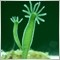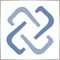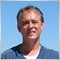• Get access

# Counting symbols and auto MagicNumber289

hi, can I use this functions in Expert Advisor Script for counting symbols?
```int curencies() {
int aa = 0, bb = 0, Sn = 0;
if(!GBPUSD) aa=1;
if(!EURUSD) bb=1;
Sn=aa+bb; return(Sn);}```
also different MagicNumber for different Periods..
`int number() { int id = Period(); return ( id ); }`
also to calculate the time ...
``` int gmttime = LocalTime() + GMT_Hour * 3600 + GMT_Minute * 60;
if ( TimeHour ( gmttime ) > 7 ) Function();```
and most important, if this function will give correct values for Points in Expert Advisor Script, as I know it would give in indcator, but in EA I don't know way to check this as somewhere I am getting Zerro Devide as error 4013?
`double Stops = MathRound((High-Low+High-Low)/Point);`
Any help would be apreciated.
Thanks.289

OK I found the 4013 error, did not noticed missed ... =0
```int value; // incorrect
int value = 0; // corrected```101289

Tatyana: